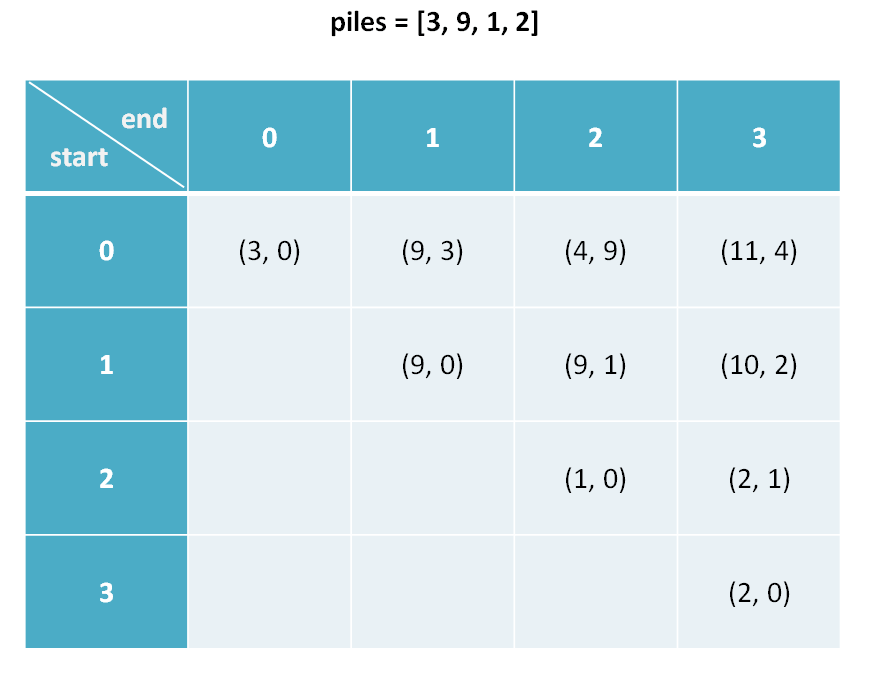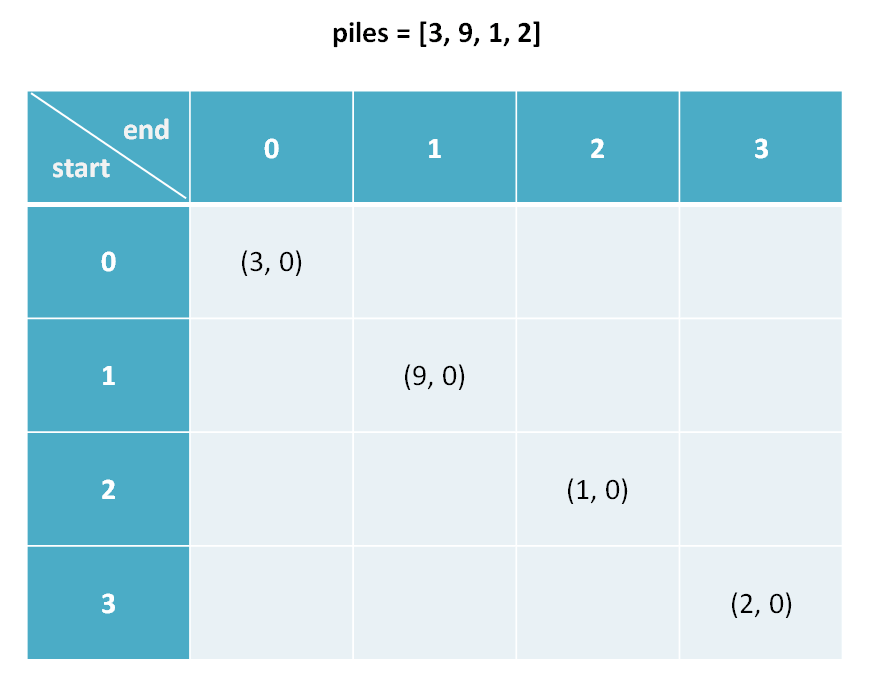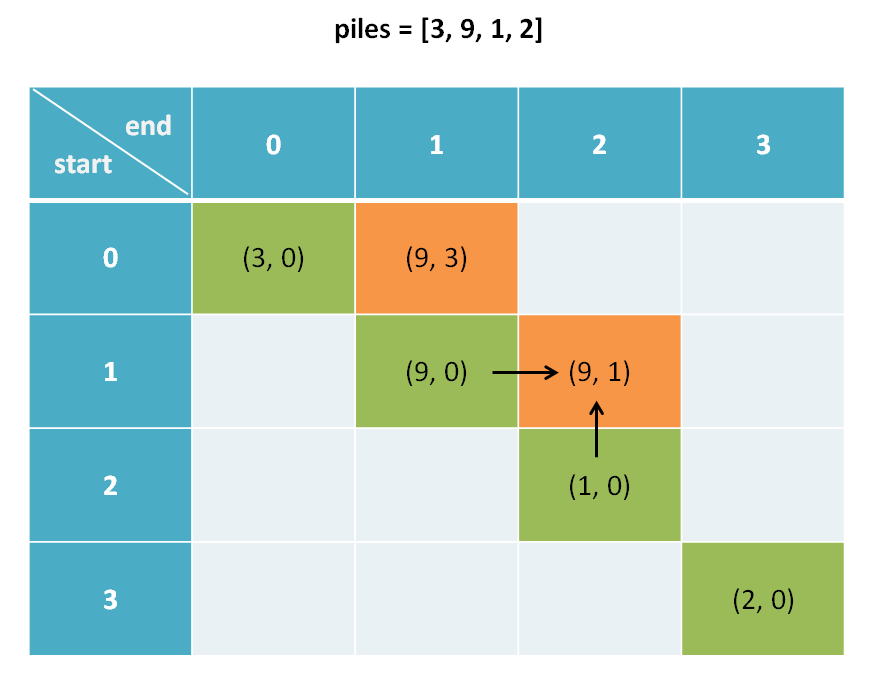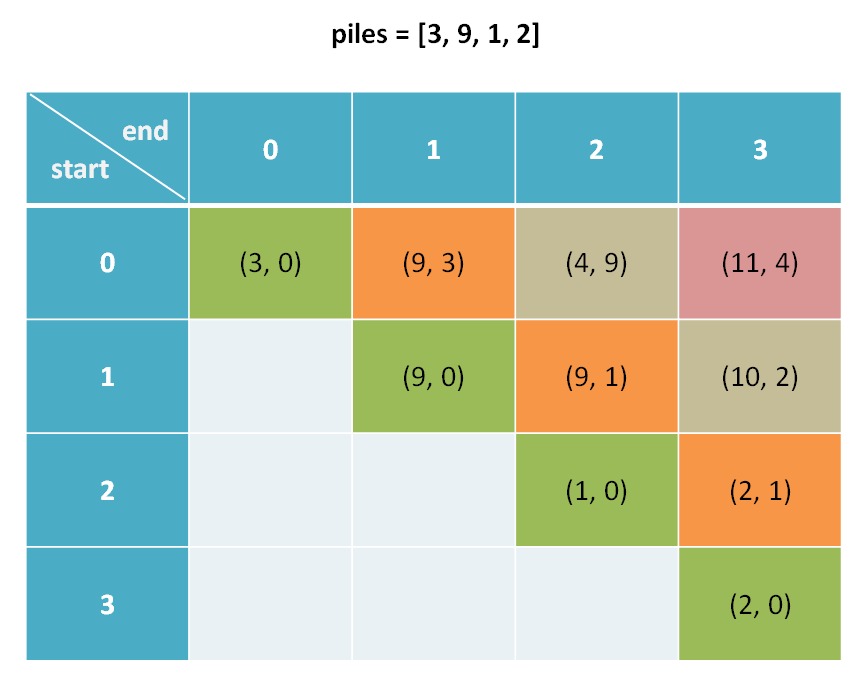# 博弈问题--石头游戏(动态规划)

## 解析思路

### 定义 dp 数组的含义dp[i][j].fir 表示，对于 piles[i...j] 这部分石头堆，先手能获得的最高分数。
dp[i][j].sec 表示，对于 piles[i...j] 这部分石头堆，后手能获得的最高分数。

dp.fir = 9 意味着：面对石头堆 [3, 9]，先手最终能够获得 9 分。
dp.sec = 2 意味着：面对石头堆 [9, 1, 2]，后手最终能够获得 2 分。

### 状态转移方程

dp[i][j][fir or sec]

0 <= i < piles.length
i <= j < piles.length

n = piles.length
for 0 <= i < n:
for j <= i < n:
for who in {fir, sec}:
dp[i][j][who] = max(left, right)

dp[i][j].fir = max(piles[i] + dp[i+1][j].sec, piles[j] + dp[i][j-1].sec)
dp[i][j].fir = max(    选择最左边的石头堆     ,     选择最右边的石头堆     )
# 解释：我作为先手，面对 piles[i...j] 时，有两种选择：
# 要么我选择最左边的那一堆石头，然后面对 piles[i+1...j]
# 但是此时轮到对方，相当于我变成了后手；
# 要么我选择最右边的那一堆石头，然后面对 piles[i...j-1]
# 但是此时轮到对方，相当于我变成了后手。

if 先手选择左边:
dp[i][j].sec = dp[i+1][j].fir
if 先手选择右边:
dp[i][j].sec = dp[i][j-1].fir
# 解释：我作为后手，要等先手先选择，有两种情况：
# 如果先手选择了最左边那堆，给我剩下了 piles[i+1...j]
# 此时轮到我，我变成了先手；
# 如果先手选择了最右边那堆，给我剩下了 piles[i...j-1]
# 此时轮到我，我变成了先手。

dp[i][j].fir = piles[i]
dp[i][j].sec = 0

# 解释：i 和 j 相等就是说面前只有一堆石头 piles[i]
# 那么显然先手的得分为 piles[i]
# 后手没有石头拿了，得分为 0这里需要注意一点，我们发现 base case 是斜着的，而且我们推算 dp[i][j] 时需要用到 dp[i+1][j] 和 dp[i][j-1]：所以说算法不能简单的一行一行遍历 dp 数组，而要斜着遍历数组：（其实还可以倒着遍历说实话，斜着遍历二维数组说起来容易，你还真不一定能想出来怎么实现，不信你思考一下？这么巧妙的状态转移方程都列出来了，要是不会写代码实现，那真的很尴尬了。

## 代码

class Pair {
int fir, sec;
Pair(int fir, int sec) {
this.fir = fir;
this.sec = sec;
}
}

/* 返回游戏最后先手和后手的得分之差 */
int stoneGame(int[] piles) {
int n = piles.length;
// 初始化 dp 数组
Pair[][] dp = new Pair[n][n];
for (int i = 0; i < n; i++)
for (int j = i; j < n; j++)
dp[i][j] = new Pair(0, 0);
// 填入 base case
for (int i = 0; i < n; i++) {
dp[i][i].fir = piles[i];
dp[i][i].sec = 0;
}
// 斜着遍历数组
for (int l = 2; l <= n; l++) {
for (int i = 0; i <= n - l; i++) {
int j = l + i - 1;
// 先手选择最左边或最右边的分数
int left = piles[i] + dp[i+1][j].sec;
int right = piles[j] + dp[i][j-1].sec;
// 套用状态转移方程
if (left > right) {
dp[i][j].fir = left;
dp[i][j].sec = dp[i+1][j].fir;
} else {
dp[i][j].fir = right;
dp[i][j].sec = dp[i][j-1].fir;
}
}
}
Pair res = dp[n-1];
return res.fir - res.sec;
}

## 总结

posted on 2020-04-26 23:35  反光的小鱼儿  阅读(553)  评论(0编辑  收藏  举报